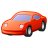# QlikView App Dev

Discussion Board for collaboration related to QlikView App Development.

Announcements
Our next Qlik Insider session will cover new key capabilities. Join us August 11th REGISTER TODAY
cancel
Showing results for
Did you mean:Contributor II

## Previous Months Data

Hello All,

I hope someone can help with my issue I'm currently experiencing.

Basically, what I want to happen is, when someone selects a month, the previous 11 months of activity are brought into 11 text boxes (one for each previous month).

I'm curently testing this out with just one month and I'm getting no joy.  This is the formula I am using within the textbox that represents the first previous month:

=Sum({\$<[Year]={\$(=Only([Year]))}, [Month] = {\$(=Only([Month])-1)} >} Activity)

I've searched high and low on this forum but the solutions I have tried have not brought me any joy.  Any help is much appreciated.

1 Solution

Accepted SolutionsLuminary Alumni

Hi,

Try this expression

=Sum({\$<[Year]={\$(=Only([Year]))}, SMonthName=, [Month] = {\$(=Only([Month])-1)} >} Activity)

Regards,

Jagan.

18 RepliesNot applicable

try this

=Sum({\$< Datefield = {\$(Vprevmonth)} >} Activity)

Variable:MVP

=SUM({\$<Year = {'\$(=Year(Today()))'}, Month = {'\$(=Month(Today())-1)'}>}Activity)Specialist

Yes What Nirmal is said is correct now copy paste the expression for Frist text box which show previos month and now for each month text box do   Sum({\$< Datefield = {\$(Vprevmonth-1)} >} Activity) for second text box and again Sum({\$< Datefield = {\$(Vprevmonth-2)} >} Activity) and so on so now when you select one year and particular month each text box shows previous month activity

Regards,

anantContributor II
Author

I can't seem to get this formula to work Manish.  Any idea why???MVP

Try This

=SUM({\$<Year = {'\$(=Year(Today()))'}, Month =  {'\$(=Date(AddMonths(Today(),-1),'MMM'))'>}Activity)Contributor II
Author

Hmm, it's highlighting the MMM part of the formula as if it's in error.  And when I put that into the textbox it returns "-".MVP

=SUM({<Year = {'\$(=Year(Today()))'}, Month = {"\$(=Date(AddMonths(Today(),-1),'MMM'))"}>}Activity)Contributor II
Author

Still not working unfortunately.  It turns everything inbetween the quote marks a redish-purple colour, as if it's a field.MVP

if your Month is in Number format below should work

=SUM({\$<Year = {'\$(=Year(Today()))'}, Month = {'\$(=Month(Today())-1)'}>}Activity)

if your Month is in Text Format below should work

=SUM({<Year = {'\$(=Year(Today()))'}, Month = {"\$(=Date(AddMonths(Today(),-1),'MMM'))"}>}Activity)

Considering that Month is 'MMM' format..

If none of above is working, let us have your sample file. There should be some Formatting issues..Community Browser